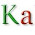Monday, 26 January 2015

Fast generation of all permutations

This current algorithm only works for odd numbers of elements. Here's the code in Java:

public static void main(String[] args)
{
int n=11;
int a,b,c,i,tmp;
int end=(int)Math.floor(n/2);
int[][] pos = new int[end+1];
int[] perm = new int[n];

for(i=0;i<n;i++) perm[i]=i;

while(true)
{
//this is where you can use the permutations (perm)
i=0;
c=n;

while(pos[i]==c-2 && pos[i]==c-1)
{
pos[i]=0;
pos[i]=0;
i++;
c-=2;
}

if(i==end) System.exit(0);

a=(pos[i]+1)%c+i;
b=pos[i]+i;

tmp=perm[b];
perm[b]=perm[a];
perm[a]=tmp;

if(pos[i]==c-1)
{
pos[i]=0;
pos[i]++;
}
else
{
pos[i]++;
}
}
}

It works by swapping the elements i and i+1 mod n, adding 1 to i after every swap. After doing this process n*(n-1) times, you loop back to the first permutation. This is where you apply the recursive step: ignore the first and last elements of the array and start the same process. After one swap on this smaller array you get back to the whole array and re-do the n*(n-1) swaps. Then you return to the smaller array and apply the next swap. After (n-2)*(n-3) swaps to the truncated array it will also loop back to its original order, this is why the algorithm is recursive, you must ignore the first and last elements of the smaller array to get a smaller one and apply the algorithm again.

Here's an example using 5 elements, keep in mind it only goes two levels deep in recursion.

0 1 2 3 4
1 0 2 3 4
1 2 0 3 4
1 2 3 0 4
1 2 3 4 0
0 2 3 4 1
2 0 3 4 1
2 3 0 4 1 ... etc.

You keep doing that till you loop to 0 1 2 3 4, here's the permutation before:

4 1 2 3 0

You'd permute 4 and 0, but instead you go down one level in recursion: you ignore 4 and 0, you permute 1 and 2.

4 2 1 3 0

Then you return to the whole array and do the process again:

2 4 1 3 0
2 1 4 3 0
2 1 3 4 0
... etc

And when you get to 0 2 1 3 4 the next step would be to swap 0 and 4 to get back to 4 2 1 3 0, instead of doing this we return to the smaller array of three elements. But now it's the second time we're here so we permute these elements to get:

0 2 3 1 4

And you go back to the full array and do those 19 steps again. In short, these are the positions you swap each time after you do the 19 steps (the 20th swap would get you to a permutation you already generated):

(...19 swaps...)
X X X X X
(...19 swaps...)
X X X X X
(...19 swaps...)
X X X X X
(...19 swaps...)
X X X X X
(...19 swaps...)
X X X X X

It's always the same process that you apply, ignoring two elements for each level of recursion you are in.

I will work on the algorithm later to optimize it and fix the issue for even numbers of elements. I will also work on a proof that it generates distinct permutations.

1 comment:

1.Very fast if it can permute 11 elements 0.39 seconds.
I use SteinhausJohnsonTrotter algorithm for 11 elements about 4 minutes.
Can you convert it to Python, please....